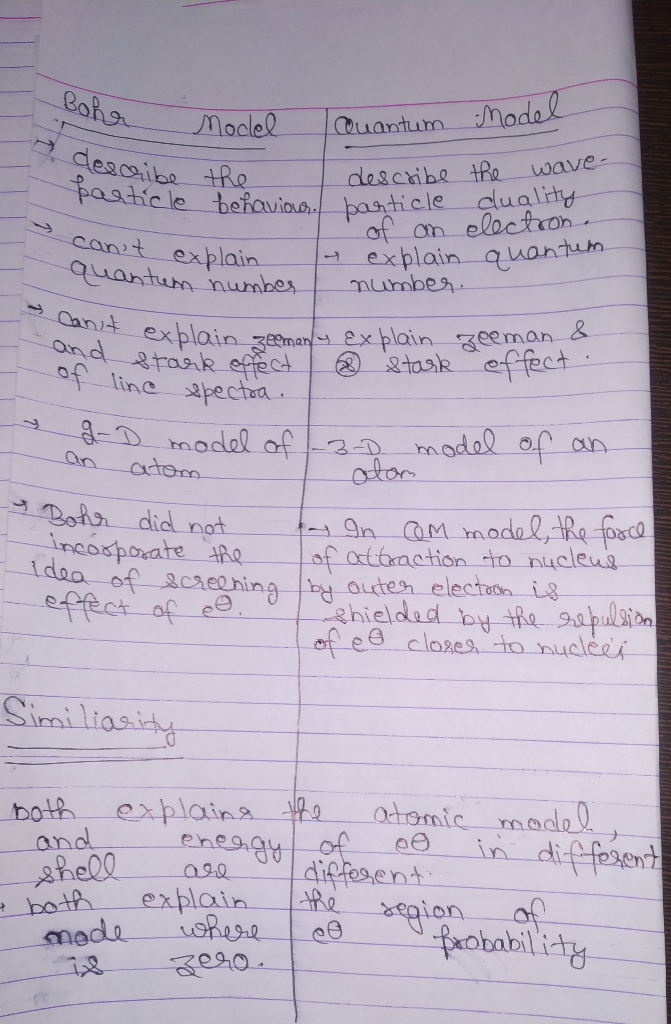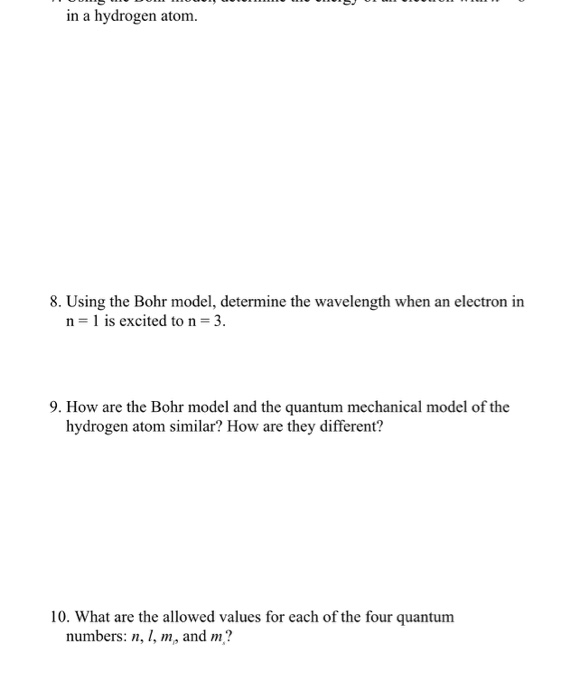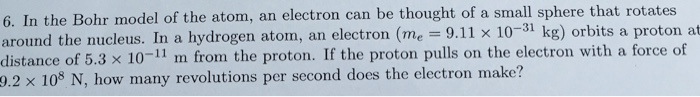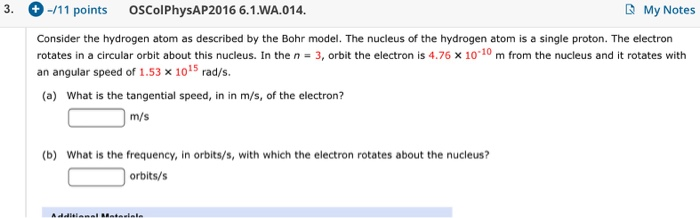Question

The structure of an atom of hydrogen can be described by the Bohr Model as well as the Quantum Mechanical Model. How are these descriptions similar? How are these descriptions different?#### Earn Coins

Coins can be redeemed for fabulous gifts.

Similar Homework Help Questions
• ### in a hydrogen atom. 8. Using the Bohr model, determine the wavelength when an electron in...in a hydrogen atom. 8. Using the Bohr model, determine the wavelength when an electron in n=1 is excited to n = 3. 9. How are the Bohr model and the quantum mechanical model of the hydrogen atom similar? How are they different? 10. What are the allowed values for each of the four quantum numbers: n, l, m, and m?

• ### Identify the orbits in the Bohr Model of the Hydrogen atom responsible for each quantum state....

Identify the orbits in the Bohr Model of the Hydrogen atom responsible for each quantum state. The Bohr Model provides that the radius of the electron’s orbit is given by: r = 0.529 x n 2 [Angstroms] (Eq. 8) where n is the state’s quantum number. Calculate the radius of each of these orbits.

• ### In lab we studied the Bohr model of the hydrogen atom which is verified exactly with...

In lab we studied the Bohr model of the hydrogen atom which is verified exactly with quantum mechanical calculations. From quantum mechanics we also find that Bohr’s equation can be used for any one-electron cation like He+, Li2+, Be3+ etc, by including the atomic number, Z, of the cation in the equation with Bohr’s constant (): En= -Z2n2(Accurate for any one-electron cation with atomic number Z) Use this equation to calculate the energy (J) of the first and second energy...

• ### Answer the following questions relating to the Bohr model of a hydrogen atom. (Be careful with...

Answer the following questions relating to the Bohr model of a hydrogen atom. (Be careful with the sign of your answers). (a) What is the energy of the n = 4 level of a H atom? J (b) What is the energy of the n = 8 level of a H atom? J (c) What is ?E for the n = 4 to n = 8 transition? J #2. Determine the energy of an electron in the given quantum level...

• ### In the Bohr model of the atom, an electron can be thought of a small sphere...In the Bohr model of the atom, an electron can be thought of a small sphere that rotates around the nucleus. In a hydrogen atom, an electron (me=9.11 x 10^-31 kg) orbits a proton at a distance of 5.3 x 10^-11 m from the proton. If the proton pulls on the electron with a force of 9.2 x 10^8 N, how many revolutions per second does the electron make? 6. In the Bohr model of the atom, an electron can...

• ### 3. -/11 points OSColPhysAP2016 6.1.WA.014. My Notes Consider the hydrogen atom as described by the Bohr model. The...3. -/11 points OSColPhysAP2016 6.1.WA.014. My Notes Consider the hydrogen atom as described by the Bohr model. The nucleus of the hydrogen atom is a single proton. The electron rotates in a circular orbit about this nucleus. In the n = 3, orbit the electron is 4.76 x 10-10 m from the nucleus and it rotates with an angular speed of 1.53 x 10"rad/s. (a) What is the tangential speed, in in m/s, of the electron? m/s (b) What is...

• ### According to the Bohr model, the energy of the hydrogen atom is given by the equation:...

According to the Bohr model, the energy of the hydrogen atom is given by the equation: E = (-21.7 x 10 -19 J)/ n 2 Calculate the wavelength of the photon emitted when the atom undergoes relaxation from the first excited state to the ground state The answer is 1.22 x 10-7 m but I don't know how ?

• ### In the Bohr model of the hydrogen atom, the allowed orbits of the electron (labeled n...

In the Bohr model of the hydrogen atom, the allowed orbits of the electron (labeled n = 1, 2, 3, …) have angular momentum , orbital radii , and energies . In these expressions me is the mass of the electron. In an exotic atom the electron is replaced by a different subatomic particle that has the same charge as an electron but a different mass. Two examples that have been studied are muonic hydrogen, in which the electron is...

• ### In the Bohr model of the hydrogen atom, the allowed orbits of the electron (labeled n...

In the Bohr model of the hydrogen atom, the allowed orbits of the electron (labeled n = 1, 2, 3, …) have angular momentum , orbital radii , and energies . In these expressions me is the mass of the electron. In an exotic atom the electron is replaced by a different subatomic particle that has the same charge as an electron but a different mass. Two examples that have been studied are muonic hydrogen, in which the electron is...

• ### A hydrogen atom is in its n = 5 state. Part A In the Bohr model,...

A hydrogen atom is in its n = 5 state. Part A In the Bohr model, what is the ratio of its kinetic energy to its potential energy?